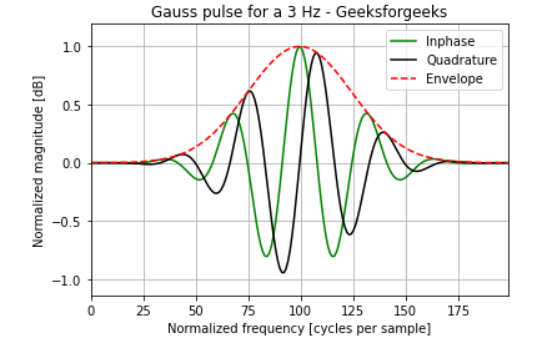# Create a gauss pulse using scipy.signal.gausspulse

• Last Updated : 03 Jan, 2021

Prerequisites: Scipy

The impulse response of a Gaussian Filter is written as a Gaussian Function as follows:

Attention geek! Strengthen your foundations with the Python Programming Foundation Course and learn the basics.

To begin with, your interview preparations Enhance your Data Structures concepts with the Python DS Course. And to begin with your Machine Learning Journey, join the Machine Learning - Basic Level CourseIts result is also Gaussian. In this article, we will plot the gauss pulse at 3Hz using scipy and matplotlib Python library. Gauss pulse is used in digital filters for motion analysis.  To create a gauss pulse scipy’s gausspulse() method is used. gausspulse() returns a unit-amplitude Gaussian-modulated sinusoidal pulse at the times indicated in array t, with a center frequency ‘fc’ in hertz(Hz).

Parameter:

• t: Input array.
• fc: Center frequency.
• retquad: If True, return the imaginary as well as the real part of the signal. Default is False.
• retenv: If True, return the envelope of the signal. Default is False.

Returns

• yI: The real part of the signal. Always returned.
• yQ:  Imaginary part of signal. Only returned if retquad is True.
• yenv:  The envelope of the signal. Only returned if retenv is True.

### Approach:

• Import required module.
• Create an array of data using np.linespace.
• Create a gauss pulse at 3 Hz.
• Label the Graph.
• Show the resultant Graph.

Implementation using the above approach is depicted below:

## Python3

 from scipy import signalimport matplotlib.pyplot as pltimport numpy as np  t = np.linspace(-1, 1, 200)  i, q, e = signal.gausspulse(t, fc=3, retquad=True, retenv=True)  plt.plot(i, color='green')plt.plot(q, color='black')plt.plot(e, '--', color='red')  plt.title('Gauss pulse for a 3 Hz - Geeksforgeeks')plt.ylabel("Normalized magnitude [dB]")plt.xlabel("Normalized frequency [cycles per sample]")plt.margins(0, 0.1)plt.grid(which='both', axis='both')plt.legend(['Inphase', 'Quadrature', 'Envelope'])  plt.show()

Output:My Personal Notes arrow_drop_up